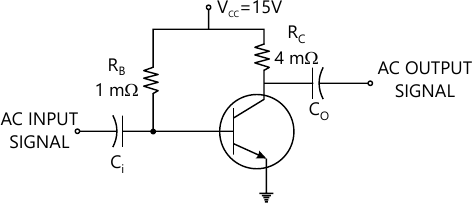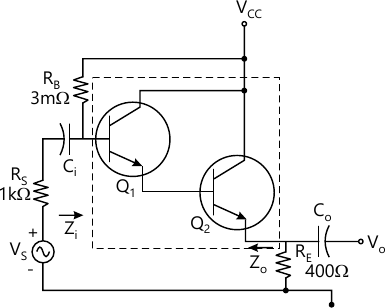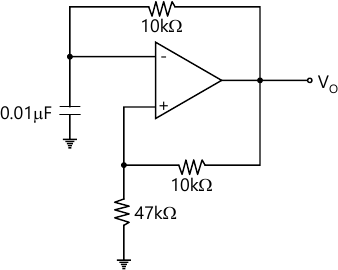MORE IN Analog and Digital Electronics
VTU Computer Science (Semester 3)
Analog and Digital Electronics
June 2014
Total marks: --
Total time: --
INSTRUCTIONS
(1) Assume appropriate data and state your reasons
(2) Marks are given to the right of every question
(3) Draw neat diagrams wherever necessary

1 (a) Draw a self bias circuit using BJT and derive the expression for operating point. Mention its advantages and disadvantage.
8 M
1 (b) For the circuit shown in Fig.Q1(b), determine the operating point. Given β;=100, VBE=0.7 V.4 M
1 (c) Explain the construction and operating principle of uni junction transistor (UJT) with relevant sketches.
8 M

2 (a) Explain the construction, working and characteristics of N-channel E-MOSFET with neat sketches.
10 M
2 (b) Given a comparison between JFETs and MOSFETs (any four).
4 M
2 (c) Briefly discuss the basic operation of CMOS inverter with a neat diagram. Mention any two advantages.
6 M

3 (a) With a neat diagram, explain the working of a photo conductor. Show how resistance varies with illuminance. Draw any two application circuits.
10 M
3 (b) What is an optocoupler? Explain the parameters of optocoupler.
6 M
3 (c) A photodiode has a noise current of 1 × 10-15 A, responsivity of 0.5 A/W, active area of 1mm2 and rise time of 3.5ns. Determine its (i) NEP; (ii) Detectivity; (iii) D*; (iv) Quantum efficiency at 850nm.
4 M

4 (a) Obtain the expression for current gain, input impedence, voltage gain and output admittance of a transistor amplifier using complete h-parameter model.
12 M
4 (b) Fig.Q4(b) shows a Darlington amplifier. The two transistors Q1 and Q2 are identical and the h-parameter for both the transistors are hic=1 KΩ , hfc=1 KΩ and hoc=40 × 10-6 mhos. THe values of voltages Vcc=15V, VBE1=0.7V and VBE2=0.7V. Determine the following:
(i) Input impedance; (ii) Output impedance; (iii) VOltage gain; (iv) Current gain.8 M

5 (a) Derive the expression for voltage gain, input resistance and output resistance in a voltage series feedback topology.
10 M
6 M
5 (c) Derive an expression for gain of an amplifier with feedback in terms of gain without feedback.
4 M

6 (a) Explain the operation of monostable multivibrator with a neat diagram.
8 M
6 (b) Explain RC low pass circuit and discuss the behaviour of this circuit towards step and pulse inputs.
8 M
6 (c) Write a note on Barkhausen criterion.
4 M

7 (a) Explain the operation of buck regulator with a neat diagram.
10 M
7 (b) Design a power transformer with a multi-output secondary and the following input/output specifications:
I. Primary voltage: 220V, 50Hz.
II. Secondary voltage: i) 12-0-12V at 100 mA and ii) 5V at 1A.
Assume B=60,000 lines per square inch and an efficiency of 90%.
6 M
7 (c) Define load regulation and line regulation of regulated power supply.
4 M

8 (a) List and explain the performance parameter of operational amplifiers.
8 M
8 (b) Explain the working of comparators as zero crossing detectors.
6 M
8 (c) For the relaxation oscillator circuit shown in Fig. Q8(c).determine the heat to heat amplitude and frequency of the square wave output given that saturation output voltage of the opamp is ± 12.5 V at power supply voltage of ± 15V.6 M

More question papers from Analog and Digital Electronics